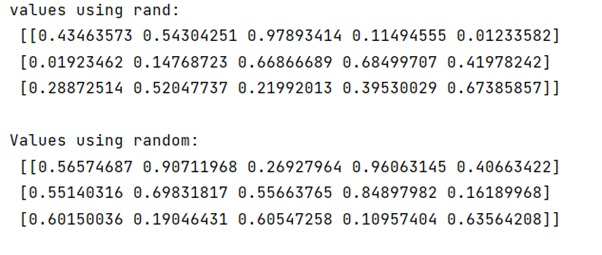# numpy.random.rand() vs numpy.random.random() Methods

Learn about the numpy.random.rand() and numpy.random.random() methods with their differences and examples in Python.
Submitted by Pranit Sharma, on February 10, 2023

NumPy is an abbreviated form of Numerical Python. It is used for different types of scientific operations in python. Numpy is a vast library in python which is used for almost every kind of scientific or mathematical operation. It is itself an array which is a collection of various methods and functions for processing the arrays.

## numpy.random.rand() vs numpy.random.random()

The numpy.random.rand() generates random values in a given shape. It creates an array of the given shape and populate it with random samples from a uniform distribution over [0, 1).

On the other hand, numpy.random.random() return random floats in the half-open interval [0.0, 1.0).

Both functions generate samples from the uniform distribution on [0, 1). The only difference is in how the arguments are handled. With numpy.random.rand(), the length of each dimension of the output array is a separate argument. With numpy.random.random_sample(), the shape argument is a single tuple.

Let us understand with the help of an example,

## Python code to demonstrate the examples of numpy.random.rand() and numpy.random.random() methods

```# Import numpy
import numpy as np

# Using random.rand

print("values using rand:\n",np.random.rand(3, 5),"\n")

print("Values using random:\n",np.random.random_sample((3, 5)),"\n")
```

Output: